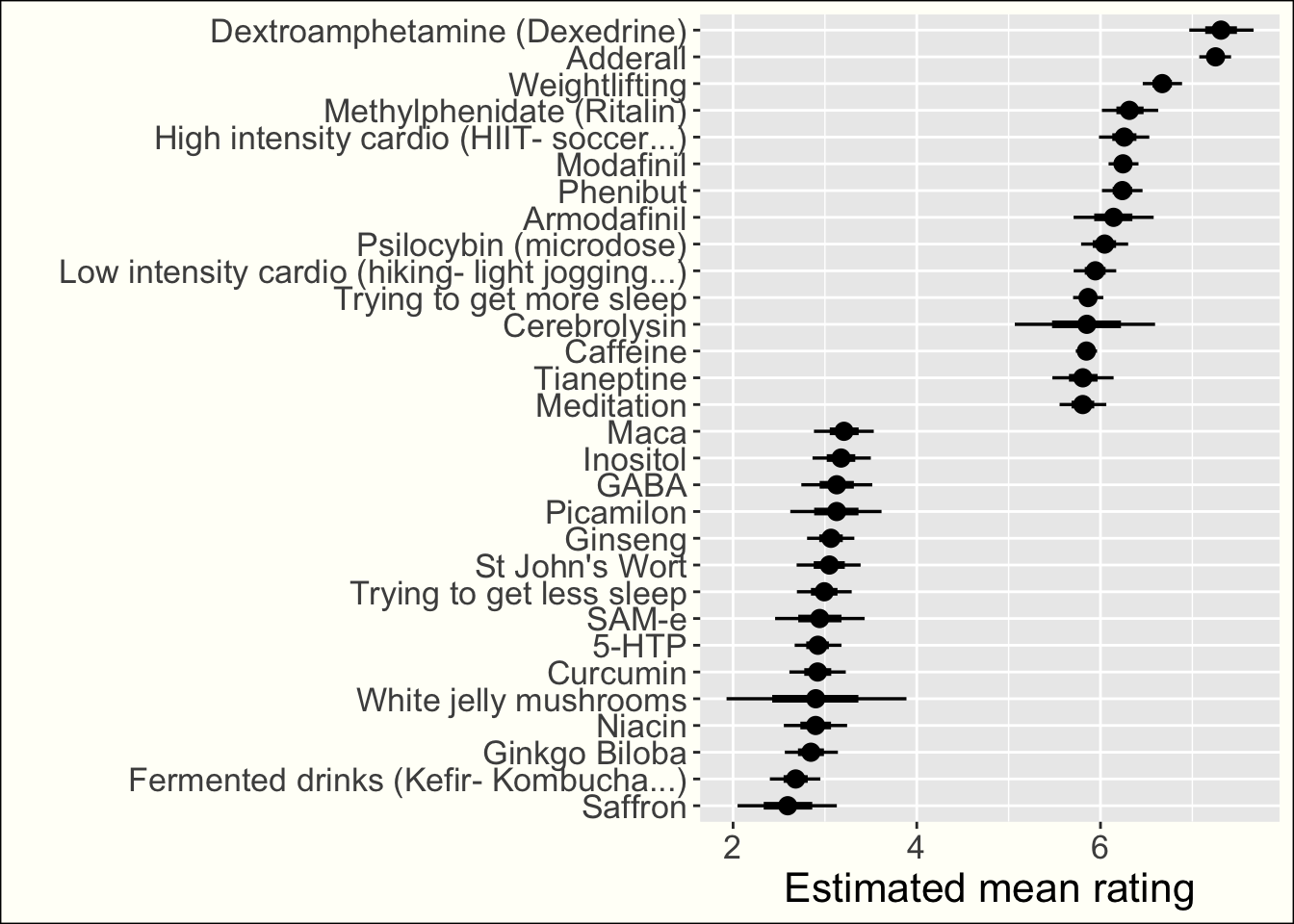``knitr::opts_chunk\$set(echo = TRUE, warning = FALSE, message = FALSE)``
``````library(tidyverse)
library(rstanarm)
library(tidybayes)
options(mc.cores = parallel::detectCores())
theme_set(theme(plot.background = element_rect(fill="#fffff8"), #to incorporte into the main article
text = element_text(size = 16)))``````
``````df <- read_csv("../data/nootroflix_ssc_ratings_clean.csv") %>%
mutate(itemID = str_replace_all(itemID, ",", "-")) # prevent bug``````

# Aggregated nootropics effect

## First variant: estimating the mean rating for each nootropic

To adjust for the fact that different users might have different rating scales, we fit a Bayesian multilevel linear regression (with default weakly informative priors): each user and each nootropic gets his own intercept (more or less close the the mean intercept, depending on the quantity of data). What we’re interested in in the intercept for each nootropic.

``````l <- stan_glmer(rating ~ (1 | itemID) + (1 | userID), data = df,
``````saveRDS(l, "saved_models/mean_ratings")
to_plot <- l %>%
filter(str_detect(group, "itemID:")) %>%
mutate(group = str_remove(group, "itemID:")) %>%
median_qi(condition_mean = `(Intercept)` + b, .width = c(.95, .66)) %>%
mutate(nootropic = group, estimated_mean_rating = condition_mean) %>%
mutate(nootropic = str_replace_all(nootropic, "_", " ")) %>%
mutate(nootropic = fct_reorder(nootropic, condition_mean))``````
``````to_plot %>%
filter(rank(estimated_mean_rating) < min(rank(estimated_mean_rating)) + 30 | rank(estimated_mean_rating) > max(rank(estimated_mean_rating)) - 30) %>%
#filter(group %in% (to_plot %>% filter(.width==0.95) %>% filter(.upper > 4.5))\$group) %>%
ggplot(aes(y = nootropic, x = estimated_mean_rating, xmin = .lower, xmax = .upper)) +
geom_pointinterval() +
xlab("Estimated mean rating") +
ylab("")````````````ggsave("plots/ratings_mean.jpeg", width=10, height=10, units = "in", limitsize = F, dpi=300)

plot_full <- to_plot %>%
#filter(group %in% (to_plot %>% filter(.width==0.95) %>% filter(.upper > 4.5))\$group) %>%
ggplot(aes(y = nootropic, x = estimated_mean_rating, xmin = .lower, xmax = .upper)) +
geom_pointinterval() +
xlab("Estimated mean rating") +
ylab("")

ggsave("plots/ratings_mean_full.jpeg", width=10, height=50, units = "in", limitsize = F, dpi=300, plot=plot_full)

#ggsave("ratings_mean_low.jpeg", width=10, height=20, units = "in", limitsize = F, dpi=100)``````

## Second variant: estimating the probability of positive effect for each nootropic

Given the scale we use, the estimated mean rating is not so easy to interpret. What we also do is to estimate the probablity that the effect of a nootropic is positive. For the scale used in the SSC survey and for Nootroflix, 0 corresponds to a neutral or negative effect, and higher ratings correspond to more-or-less confidence in a positive effect.

To this aim, we replace the linear regression above by a logistic regression. To make sure that our results are not biased by the people who haven’t read the scale description (and might rate a negative effect higher than zero), we say that a nootropic had a positive effect on a user if its rating was more than the user’s minimum rating (and we remove users with too few ratings).

``````l_effective <- stan_glmer(is_effective ~ (1 | itemID) + (1 | userID), data =  df %>%
group_by(userID) %>%
mutate(n_ratings = n(), min_rating = min(rating)) %>%
filter(n_ratings > 10) %>%
mutate(is_effective = if_else(rating > min_rating, 1, 0)),
``````saveRDS(l_effective, "saved_models/effective_ratings")
to_plot_effective <- l_effective %>%
filter(str_detect(group, "itemID:")) %>%
mutate(group = str_remove(group, "itemID:")) %>%
mutate(condition_mean = `(Intercept)` + b, proba = exp(condition_mean) / (1 + exp(condition_mean))) %>%
median_qi(proba, .width = c(.95, .66)) %>%
mutate(nootropic = group) %>%
mutate(nootropic = str_replace_all(nootropic, "_", " ")) %>%
mutate(nootropic = fct_reorder(nootropic, proba))

#Only best and wort
to_plot_effective %>%
filter(rank(proba) < min(rank(proba)) + 30 | rank(proba) > max(rank(proba)) - 30) %>%
#filter(group %in% (to_plot %>% filter(.width==0.95) %>% filter(.upper > 2))\$group) %>%
ggplot(aes(y = nootropic, x = proba, xmin = .lower, xmax = .upper)) +
geom_pointinterval() +
xlab("Probability of positive effect") +
ylab("")``````